# MCQs on Control Systems

##### Page 17 of 23. Go to page 1 2 3 4 5 6 7 8 9 10 11 12 13 14 15 16 17 18 19 20 21 22 23
01․ A control system has open loop transfer function G(s) = k/(s(s + 2). Find the break away point?
-1
1
-2
2

The roots of dk/ds = 0 will give the break away points. Given characteristic equation is s² + 2s +k =0 k = -s² -2s dk/ds = -2s -2 =0 Break away point s = -1

02․ Find the angles of asymptotes of the open loop transfer function G(s) = k(s + 1)/((s(s+2)(s+4)?
90°,180°
0°, 180°
90°,270°
60°,240°

Angle of asymptotes = (2q + 1)*180°/(P - Z) Given transfer function G(s) = k(s + 1)/((s(s+2)(s+4) P = 3 and Z = 1, P - Z = 2 Angle of asymptotes = 90° and 270°

03․ Angle condition for complimentary root locus or inverse root locus is
± (2q + 1) 180°
± (2q) 180°
± (2q + 1) 360°
± (2q) 360°

The angle condition is used for checking whether any point lies on root locus or not and also validity of the root locus shape. 1 + G(s)H(s) = 0 Angle condition for root locus = ± (2q + 1) 180° Angle condition for complimentary root locus = ± (2q) 180°

04․ Angle of asymptotes for complimentary root locus is
(2q+1)*180/(P-Z)
(2q+1)*180/P
(2q)*180/(P-Z)
(2q)*180/P

Shape of root locus will depends on angle of asymptotes. The "P - Z" branches will terminate at infinity along certain straight lines known as asymptotes of root locus. Angle of asymptotes for root locus = (2q+1)*180/(P-Z) Angle of asymptotes for complimentary root locus = (2q)*180/(P-Z)

05․ The characteristic equation of a control system is given below: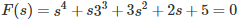The system is
stable
marginally stable
unstable
critical stable

Given characteristic equationApplying Routh-Hurwitz criterion as shown below,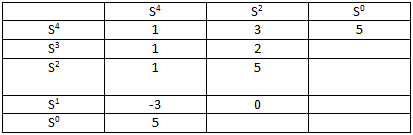There are two sign changes in the first column of the Routh array, so the system is unstable.

06․ A unity feed back system has forward transfer function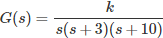The range of k for the system to be stable is
0 < k < 390.
0 < k < 39.
0 < k < 3900.
None of the above.

Characteristic equation is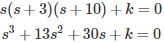Applying Routh-Hurwitz criterion as shown below,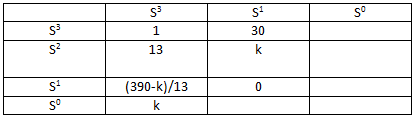For system to be stable k > 0 and 390 - k > 0. Therefore, 0 < k < 390.

07․ The open loop transfer function of a unity feedback system is given by G(s) = 1/(s+2)². The closed loop transfer function will have poles at
-2,-2
-2,-1
-2+j,-2-j
-2,2

Open loop transfer function G(s) = 1/(s+2)² For unity feedback system H(s) = 1 Therefore, closed loop transfer function = G(s)/(1 + G(s)H(s)) = 1/(s² + 4s + 5) Therefore, closed loop poles will be the roots of s² + 4s + 5 = 0 i.e. s = -2+j, -2-j

08․ A transfer function has its zero in the right half of the s-plane. The function
is positive real
is minimum phase
is non-minimum phase
will give stable impulse response

Linear time invariant system transfer functions should be minimum phase functions i.e their poles and zeros must lie in left hand side of s-plane only. Non-minimum phase functions have their zeros in the right half of the s-plane.

09․ Electrical analogous element for damper element (force-voltage analogy) in mechanical translation system is
capacitor
inductor
resistor
any of the above

The electrical equivalents of the mechanical elements is known as analogous system. The following shows the analogous quantities in Force-Voltage analogy Mechanical element ----------- Electrical analogous element
1. Mass element (M) ---------- Inductance (L)
2. Spring element (K) --------- Inverse capacitance (1/C)
3. Damper -------------- Resistance (R)
4. Velocity (v) ----------------- Current (i)
5. Displacement (x) ----------- Charge (q)
6. Force (f) --------------------- Voltage (V)

10․ When deriving the transfer function of linear element